[  HOME  ]

:  SCIENCE PARK  :

 3D Pendulum Centrifugal Force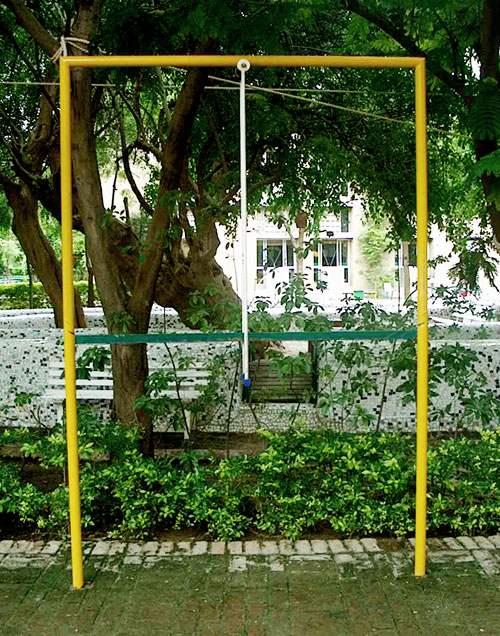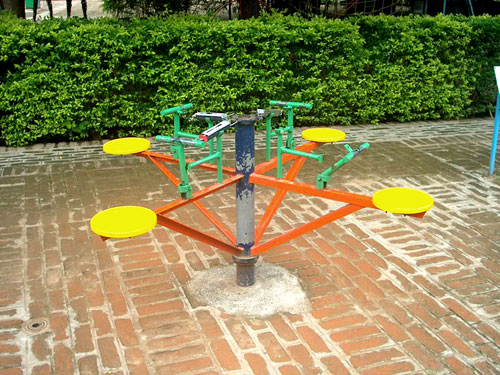There are two pendulum suspended from the top. Keep the two pendulums away from each other and leave it to oscillate freely. Now view the two pendulums through glass provided which is partly covered with a red colored film. You will get an illusion like the pendulum rotate inn a three dimensional plane. The force acting over any particle that revolves about an axis, factors governing that force are explained with fun-filled merry-go round. Circus Of Energy Cyclonical Path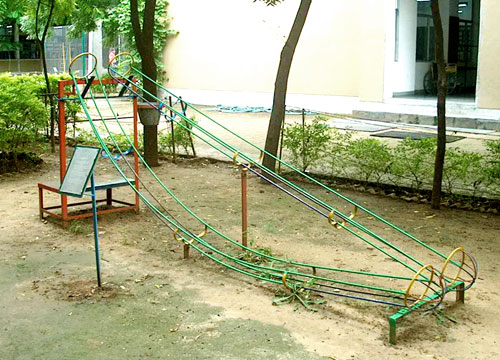The massive structure with combination of paths, explain potential energy, kinetic energy, centrifugal force, transformation of energy from one form to another and work done by spending energy. Gaining acceleration due to gravity is varied with two different shaped paths. Double Ended Cone Friction Of Speed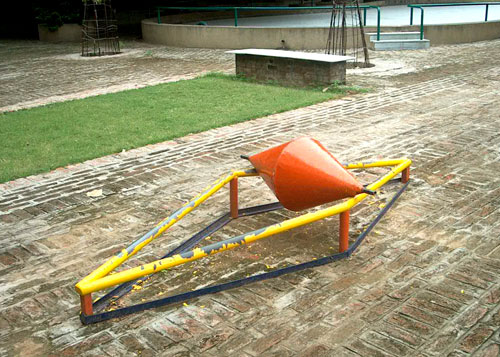The equilibrium of any object is governed by the position of it's center of gravity. This fascinating model explains it with fun. Three balls, challenging each other, teach us the relationship between friction, texture and speed. Gravity Ball Loop The Loop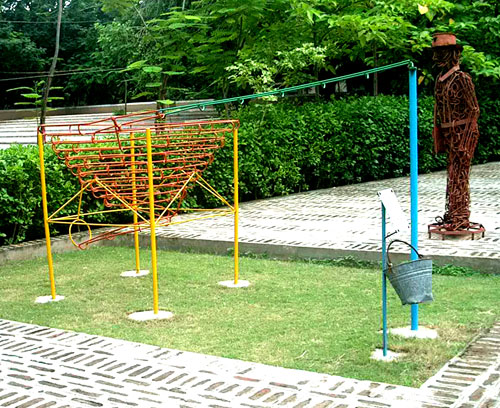Rate of change of velocity can be viewed by allowing a ball to roll here. Potential energy converted into kinetic energy and overcoming gravitational pull through centrifugal force obtained at a circular loop, all these explained here. Newton's 3rd Law Periodic Table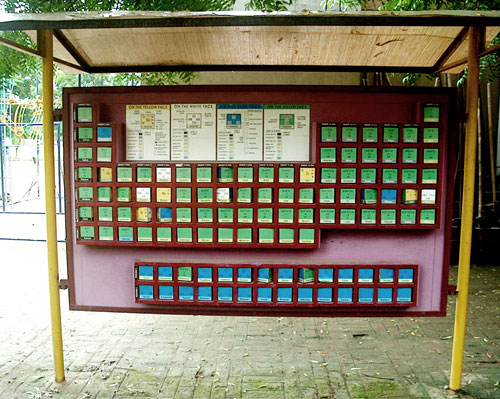Wonderful play model for explaining that,, for every action there is an equal and opposite reaction. A team of children can play quiz over this table by locating elements and their atomic number. Principle Of Lever Sympathetic Swing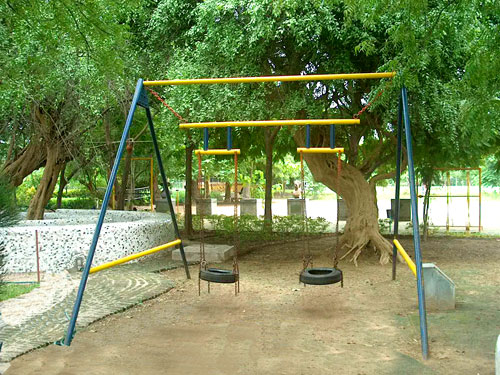The mechanical advantage gained through a first order lever is illustrated with varying fulcrum distance from weight. The resonance principle is explained with a wonderful play model here. Resonance energy transfer and perpetual motion all these are explained here. Wave Motion World Time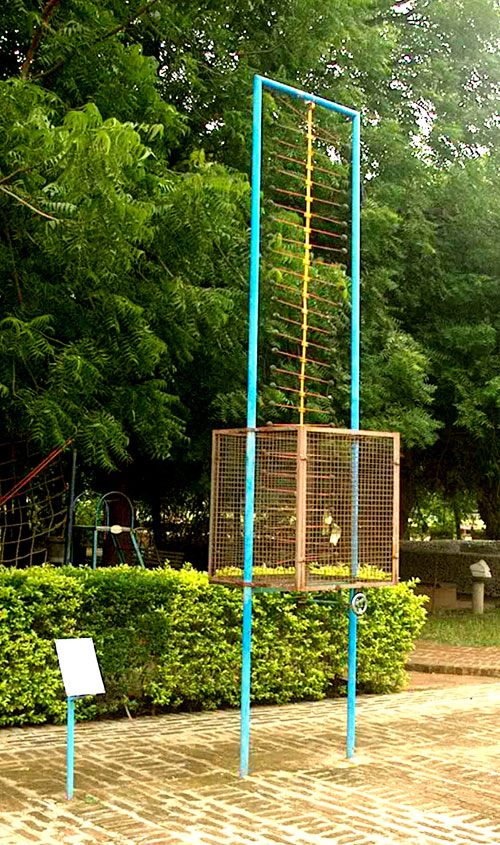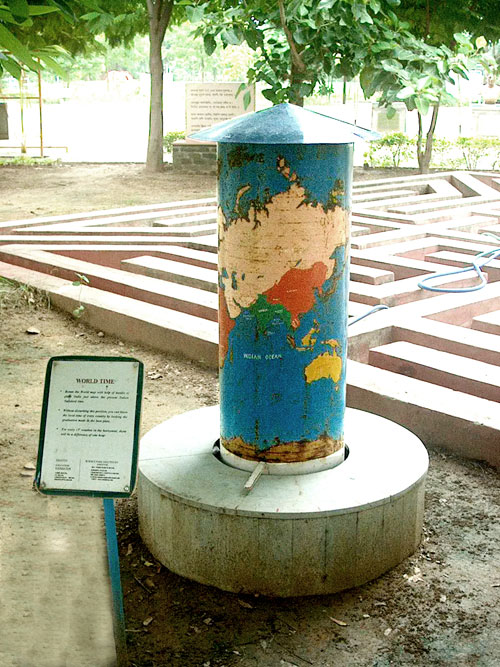Node, anti-node and collision of two waves can be visualised here. The change in local time of every country, with reference to the latitude of that country is explained here. How Friction Helps Angular Momentum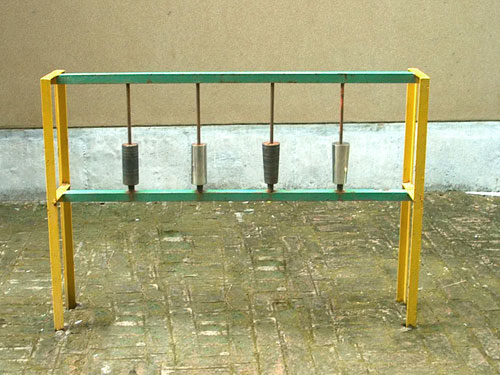Friction is more essential to move or stop. The role of friction is explained here. Concentration of mass at rotating axis, increases angular velocity and angular momentum, explained through this single seat merry-go round. Archimedes's Screw Sine Wave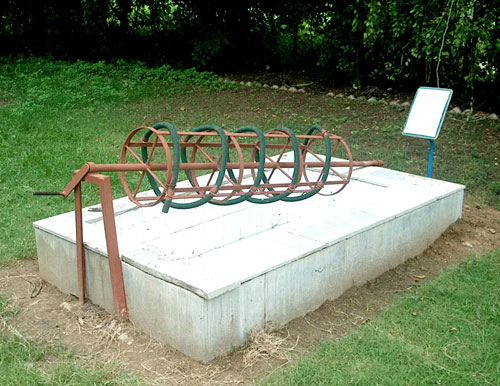Centrifugal force utilized in ancient days for water lifting is explained. Rolling ball over peaks, explains potential energy and kinetic energy well. Pulley and pulley blocks Parabolic Dishes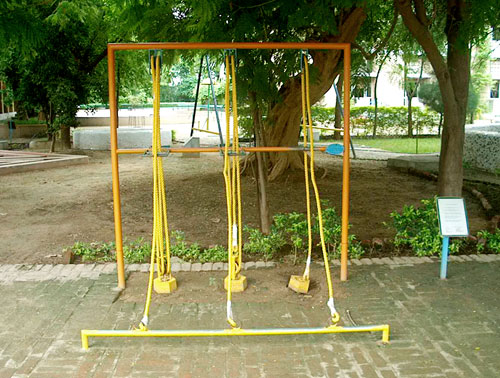The mechanical advantage gained by coupling number of pulleys explained here. Sound waves have the property similar to light like reflection, convergence and divergence. This fun filled model explains it clearly. Projectile Lissajou's Figures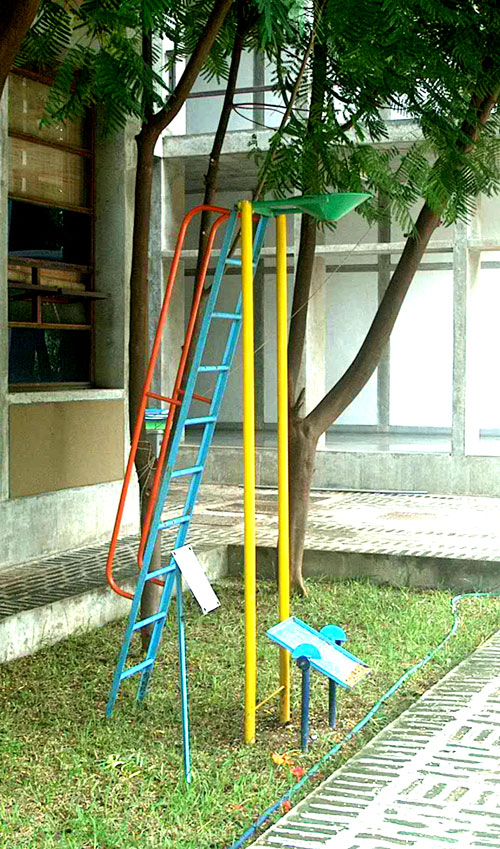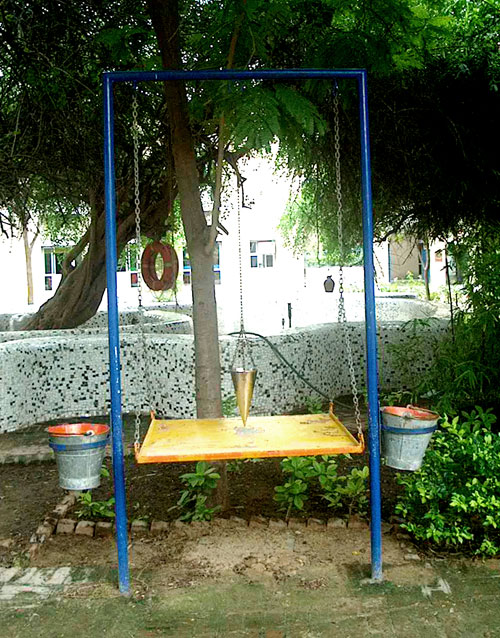Fascinating facts of trajectory is explained by this gadget. The resultant of two simple harmonic motions is explained here. Gear, belt and chain drives First Order Lever Seesaw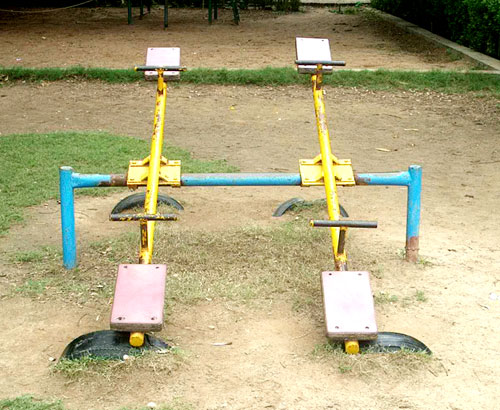Power transmitted from one axis to another is explained here with various mechanisms. With different fulcrum positions, a play model explains the leverage we gain through lever system.

 [  HOME  ]